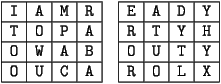# Lecture 4: Non-Mathematical Ciphers

In our last lecture, we are going to discuss some new ways to create ciphers. Unlike in the previous lectures, these ciphers depend less on mathematics. Of course, some ideas will still carry over. Anything we discuss here will work perfectly well in a more general setting, but we are no longer going to encode punctuation or digits and will not be dividing our messages into uniform blocks for ease of presentation.

### The Keyword Cipher

So far, every cipher we have considered has been a substitution cipher. That is, for each letter (or block of letters) of plaintext, we substitute a unique letter (or block of letters) to get our cipher text. Let's now consider some another substitution cipher. First, we choose a keyword, preferably one with out any repeated letters. At the end of our keyword, we write the rest of our alphabet, in order, with the letters in the keyword excluded. Aligning this with the unaltered alphabet gives the cipher. The following example should make this process clear. Suppose Alice wants to send Bob the following message using the keyword cipher with `PARTY` as the keyword:
```MAKE SURE JOE IS GONE BY SEVEN. ALICE.
```
Now, we create our ciphertext alphabet and compare to our plaintext alphabet
```plaintext:  A B C D E F G H I J K L M N O P Q R S T U V W X Y Z
ciphertext: P A R T Y B C D E F G H I J K L M N O Q S U V W X Z
```
```IPGY OSNY FKY EO CKJY AX OYWYJ. PHERY.
```
To decrypt this message, Bob needs to know that the keyword is party. Once he knows that, he swaps the position of the alphabets:
```ciphertext: P A R T Y B C D E F G H I J K L M N O Q S U V W X Z
plaintext:  A B C D E F G H I J K L M N O P Q R S T U V W X Y Z
```

### The Square Cipher

This is our first example of a cipher that is not a substitution cipher. It is created by writing our message in a series of boxes and the rearranging the boxes. It is best explained by an example. Suppose Carol wants to send Dave the following message:
```I AM READY TO PARTY! HOW ABOUT YOU? CAROL.
```
Notice that without punctuation, there are 31 characters in Carol's message. Adding a dummy `X` at the end makes 32. Now draw two four by four square, and write the message out, one letter in each box going all the way across both boxes, one row at a time:Now, to get her encrypted message, Carol writes the last column, top to bottom. Then she writes the second to last column, and so forth. Her encrypted message looks like:

```YHYX DYTL ATUO EROR RABA MPAC AOWU ITOO
```
Alice could have arranged the columns in any order she wanted to. Of course, to decrypt the message, Bob needs to know both what size square Alice used and what order in which she wrote the columns.

### The Date Cipher

The last type of cipher we are going to discuss is a mathematical cipher, but it is not a substitution cipher. In stead it is a type of shift cipher where each character is shifted by a different amount, determined by today's date. For example, suppose Alice wants to send the following message to Bob, Carol, and Dave on August 30, 2008:
```PARTY TIME!!!
```
First, she writes the numerical date (8/30/2008) over her message, repeating as often as necessary:
```83020 0883020
PARTY TIME!!!
```
Next, she shifts each character to a number and then adds to that the number written directly above, reducing each modulo 41 gives the ciphertext:
```2404182225 20172108384038
```
So long as the recipients know the date that was used to encrypt the message, it is as easy to decrypt as the shift cipher!

### Exercises

This work was made possible through a grant from the National Science Foundation.